# Mathematical Functions

Mathematical Functions are used to modify data with the Data Tools | Data | Transform command in the worksheet or create function plots in the plot window.

## Data Types

The expression evaluator supports 32-bit signed integer numbers, double-precision floating-point numbers, a Boolean value, a text string of 0 to 256 characters, and time stamp values.

## Variable Names

Variable names must begin with a column letter (i.e. A), row number (i.e. _1), or cell location (i.e. A2), which may be followed by other letters, numbers, or underscores (_), up to a maximum of 256 characters per variable name.

The variable names are not case sensitive. For example, sum(a..z), sum(A..z), and sum(A..Z) all refer to the same variable.

## Precedence

The mathematical expression can consist of constants, variables (such as column letters), or functions (outlined below). The formulas follow standard precedence rules. Spaces are used in the equation for clarity.

Operators of equal precedence are evaluated from left to right within the equation. Parentheses are used to override precedence, and expressions within parentheses are performed first.

Operators, in order of decreasing precedence are:

 (  ) parentheses - minus (or negative sign) ^ exponentiation (raise-to-the-power-of) * / multiplication and division + - addition and subtraction

The expression evaluator treats operators with the following precedence:

1. !, NOT, ~

2. ^, POW

3. *, /, %

4. +, -

5. <<, >>

6. <, >, <=, >=

7. ==,!=,<>

8. &

9. XOR

10. |

11. &&, AND

12. ||, OR

13. ?:

14. IF

## Built-in Functions

The following built-in functions are supported:

### Trigonometric Functions

All trigonometric functions are carried out in radians. If the data are in degrees, use the d2r(x) conversion function (in the Miscellaneous Functions section below) to convert degree data to radians and then use the trigonometric functions.

 sin(x) sine of angle x cos(x) cosine of angle x tan(x) tangent of angle x, the value of x must not be an odd multiple of P/2. asin(x) Arcsine in the range -P /2 to P/2, x must be between -1 and 1 acos(x) Arccosine in the range 0 to P, x must be between -1 and 1 atan(x) Arctangent in the range -P/2 to P/2 atan2(y,x) Arctangent in the range -P to P

### Bessel Functions

 j0(x) j1(x) jn(n,x) Bessel functions of the first kind at x of orders 0, 1, and n, respectively y0(x) y1(x) yn(n,x) Return the Bessel functions of the second kind at x, of orders 0, 1, and n, respectively. For y0, y1, and yn, the value of x must not be negative.

### Exponential Functions

 exp(x) exponential function of x (e to the x) sinh(x) hyperbolic sine of angle x cosh(x) hyperbolic cosine of angle x tanh(x) hyperbolic tangent of angle x ln(x) natural logarithm of x, x must be positive log10(x) base 10 logarithm of x, x must be positive pow(x,y) x raised to the yth power Alternatively use x^y Error conditions result if x is zero and y is negative or zero, x is negative and y is not an integer, an overflow results.

### Miscellaneous Functions

 min(x,y) smaller of x and y max(x,y) larger of x and y randn(x,y) an approximately normally (Gaussian) distributed real random number with mean x and standard deviation y randu(x) a uniformly distributed real random number from the interval [0,x] row() row number ceil(x) smallest integer that is greater than or equal to x floor(x) largest integer less than or equal to x pi() returns the value of Pi. To limit to a specific number of digits, use Round(Pi(),y) where Y is the number of digits after the decimal point round(x,y) X rounded to the nearest number with Y digits after the decimal point sign(x) Evaluates the sign of x. Returns -1 when x < 0, returns 0 when x = 0, returns 1 when x > 0 sqrt(x) square root of x, x must not be negative fabs(x) absolute value of x fmod(x,y) floating point remainder of x/y, if y is zero, fmod returns zero d2r(x) convert argument in degrees to radians, for example: sin(d2r(30)) computes the sine of 30 degrees, sin(30) computes the sine of 30 radians r2d(x) convert argument in radians to degrees

### Statistical Functions of an Interval

 sum(a..z) calculates the sum of a range of columns in a row sum(_1.._5) calculates the sum of a range of rows in a column avg(a..z) calculates the average of a range of columns in a row avg(_1.._5) calculates the average of a range of rows in a column std(a..z) calculates the (population) standard deviation of a range of columns in a row std(_1.._5) calculates the (population) standard deviation of a range of rows in a column rowmin(a..z) finds the minimum value of a range of columns in a row rowmin(_1.._5) finds the minimum value of a range of rows in a column rowmax(a..z) finds the maximum value of a range of columns in a row rowmax(_1.._5) finds the maximum value of a range of rows in a column

The statistical functions of an interval of columns operate row-wise on an interval of columns. For example, SUM(A..Z) computes the sum of the twenty-six columns A, B, C, ..., Z separately for each row. You can replace A..Z with any valid interval of columns, e.g., C..H or W..AC. There must be exactly two periods between the column labels. Columns may be given in reverse order, i.e., SUM(Z..A).

The statistical functions of an interval of rows operate column-wise on an interval of rows. For example, SUM(_1.._5) computes the sum of the 5 rows 1, 2, 3, 4, 5 separately for each column. You can replace _1.._5 with any valid interval of rows, e.g., _3.._12 or _34.._413. There must be exactly two periods between the row labels. Rows may be given in reverse order, i.e., SUM(_5.._1).

### String Comparison

 atof(x) converts string to floating-point number atoi(x) convert a string x to an integer value ftoa(x,y) convert a floating-point number x to a string with y digits after the decimal strlen(x) length of string x in characters strcmp(x,y) compare string x with y and return 1 if x>y, -1 if x

String comparison functions work with strings, not numbers. Any rows or columns containing numbers result in blanks. In each of the string comparison functions, 1 is returned if string x is greater than string y, -1 is returned if string x is less than string y, and 0 if string x = string y. In the three-parameter comparison functions, the third parameter, z, specifies the number of characters to compare. For example, a z value of 3 compares the x and y strings' first three characters and ignores any characters after the third.

The comparisons are based on the standard ASCII table:

1.   numeric values (disregarded in string comparisons as mentioned above)

2.   cells starting with a space character

3.   common punctuation

4.   numeric text (numbers entered as text)

5.   less common punctuation

6.   uppercase letters

7.   even less common punctuation

8.   lower case letters

9.   uncommon punctuation

10.   blank cells (disregarded in string comparisons)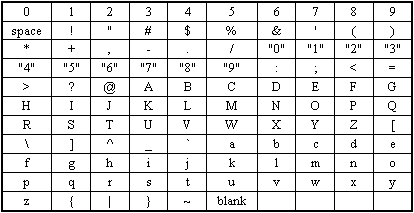This is the ASCII table order. The table is read left to right, top to bottom.
Items appearing toward the upper left corner are less than the items
appearing toward the lower left corner.

### Boolean Expressions

Boolean expressions, include:

·      logical operators (and, or, xor, not)

·      comparison operators (=, <>, <, >, <=, >=)

·      the IF function, i.e., IF(condition,true_value,false_value)

The words AND, OR, XOR, NOT, and IF are reserved keywords and may not be used as variable names.

#### Logical Operators (and, or, xor, not)

 SYMBOL NAME DESCRIPTION AND AND The result is true if both operands are true && AND The result is true if both operands are true ! Logical NOT Inverts the Boolean value. True becomes false, false becomes true NOT Logical NOT Inverts the Boolean value. True becomes false, false becomes true & AND The result is true if both operands are true | OR The result is true if either of the two operands are true XOR Exclusive-OR (XOR) The result is true only when the two operands are different || OR The result is true if either of the two operands are true OR OR The result is true if either of the two operands are true

#### Comparison Operators (=, <>, <, >, <=, >=)

 SYMBOL NAME DESCRIPTION ~ Bitwise NOT Inverts the bits in an integer * Multiple Multiplies the two operands / Divide Divides the first operand by the second % Remainder Integer remainder of the first operand divided by the second + Add Adds the two operands - Subtract Subtracts the second operand from the first << Shift Left Shifts the operand to the left >> Shift Right Shifts the operand to the right < Less Than Result is true if the value of p1 is less than the value of p2 <= Less Than or Equal To Result is true if the ordinal value of p1 is less than or equal to p2 > Greater Than Result is true if the ordinal value of p1 is greater than p2 >= Greater Than or Equal To Result is true if the ordinal value of p1 is greater than or equal to p2 == Equal To Result is true if the operands have identical values != Not Equal To Result is true if the operands do not have identical values <> Not Equal To Result is true if the operands do not have identical values

#### IF Function IF(condition, true_value, false_value)

 SYMBOL NAME EXAMPLE DESCRIPTION IF Conditional Evaluation IF(p1,p2,p3) IF(condition,true_value,false_value) If p1 is true, the result will be p2. If p1 is false, the result will be p3 IF Conditional Evaluation p1?p2:p3 condition?true_value:false_value If p1 is true, the result will be p2. If p1 is false, the result will be p3

### Examples

The following are examples of mathematical function syntax. If you use Transform in the worksheet, replace X, Y, and Z with column letters (A is column A), row numbers (_1 is row 1), or cell locations (A1).

 Equation Mathematical Function Syntax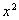x^2  OR pow(x,2)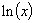ln(x)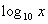log10(x)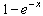(1-exp(-X))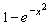1-exp(-x^2)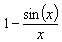1-(sin(x)/x)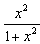x^2/(1+x^2)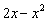(2 * X)-pow(x,2)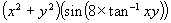(pow(x,2)+pow(y,2))*(sin(8*atan(x*y)))